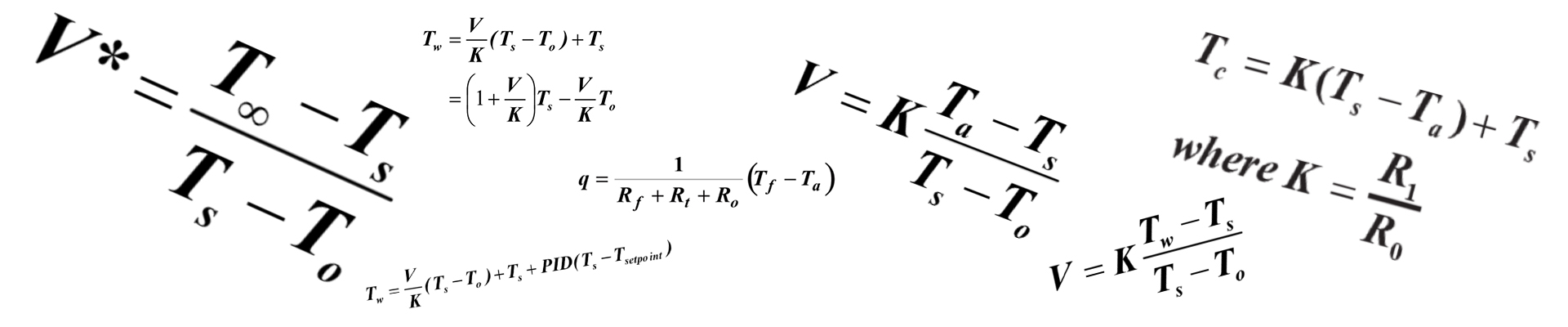# #59 HOW THE LO E FILTER REDUCES ERRORS DUE TO EMISSIVITY VARIATIONS

Emissivity is the property of a material’s surface that describes its “efficiency” at emitting thermal radiation. An emissivity value of 1.0 represents emission at 100%, and 0 describes emission at 0% (or perfect reflection - see Tech Note #31).

For non-metals and coated metals this efficiency of emission, called emissivity, is very high (0.8 or greater), and variations are usually not a problem. For example, for a production process in which a non-metallic material is to be controlled, and normal material variations cause emissivity variations of ±.01, the associated temperature error will be of the order of .01 divided by .9, or ~1% of reading, an acceptable variation. In contrast, if we are to control the temperature of a metal with emissivity 0.2, then variations of ±.01 will produce an error of the order of (.01/.2), or ~ 5% of reading. Additionally, metal finishes, which play a significant role in emissivity, tend to cause more variations than changes in finish in non-metals.

The IRt/c Lo E Filter design filters out the effects of these emissivity variations on measured temperature by approximately a factor of four, and thus reduces the errors by a factor of four. Thus, with the Lo E Filter, the errors are of the same order as those commonly experienced for high emissivity targets.

The method takes advantage of the basic physics of thermal radiation, in which the mathematical description of the energy distribution is by a formula called the Planck function:

where  is radiated energy at a given wavelength,  is the emissivity, T the absolute target temperature,  the wavelength, and the other symbols are for various physical constants. The Planck function integrates to the more familiar Stefan-Boltzman equation:

when all wavelengths are measured. The Lo E Filter works by measuring the energy content of the radiation, as described by the Planck function, over wavelengths that are more selectively sensitive for temperature variations, and therefore proportionately less sensitive to emissivity variations, as follows:

If we compute the partial derivative of each expression with respect to emissivity and temperature, we obtain the following relations for the slope of the signal with respect to temperature divided by the slope of the signal with respect to emissivity:

Accordingly, by optimum selection of the wavelengths to be measured, the sensitivity to emissivity variations can be significantly reduced, i.e. filtered, by enhancing the relative sensitivity to temperature. In practice, the best wavelengths are the shorter ones, since they provide the most sensitivity to temperature, and the least sensitivity to emissivity, as is predicted by the integration of the Planck function.

The “filtering factor” for the IRt/c Lo E models is based on the selection of .1 to 5 micron for the measured wavelengths, and results in a factor of from four to six error reduction, depending on target temperature.

As an additional benefit of the Lo E Filter, errors due to such factors as smoke, dust, moisture, etc. which may partially block the optical path to the target, are also filtered. These factors behave mathematically identically to emissivity, and therefore will be filtered by the same factor of four to six.